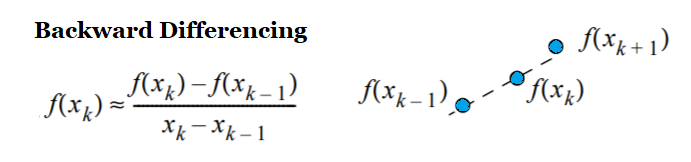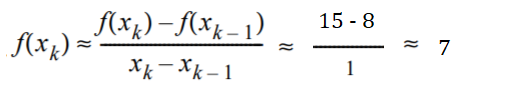# Backward Differencing

Backward differencing is a way to estimate a derivative with a range of x-values. The algorithm “moves” the points closer and closer together until they resemble a tangent line.

## Backward Differencing FormulaIf you’ve calculated slopes before, the formula might look familiar: it’s a variation on the theme. There are four parts to the formula:

• xk: The x-value you’re estimating at.
• f(xk): The function value at xk.
• xk-1: The x-value, less the step size. For example, if you’re estimating at x = 2 with a step size of 1, then xk-1 is 2 -1 = 1.
• f(xk-1): The function value at xk-1.

Example question: Approximate the derivative of f(x) = x2 + 2x at x = 3 using backward differencing with a step size of 1.

Step 1: Identify xk. This is given in the question as x = 3.

Step 2: Calculate f(xk), the function value at the given point. For this example, that’s at x = 3. Inserting that value into the formula we’re given in the question: (f(x) = x2 + 2x ), we get:
f(3) = 32 + 2(3) = 15

Step 3: Identify xk-1. This your x-value from Step 1, minus 1:
3 – 1 = 2.

Step 4: Find f(xk-1), the function value one step behind. We’re given that the step size is 1 in the example problem, so we’re calculating the value at x = 2:
f(2) = 22 + 2(2) = 8

Step 5: Insert your values into the formula and solve:## Other Types of Differencing

Other types of differencing are forward differencing, and central differencing.

• Forward differencing: When h (the distance between the two points) is greater than zero (i.e. h > 0).
• Backward differencing: When h < 0.
• Central differencing: An average of the two methods (using three points).You have to use backwards differences at the right endpoint of a function (at xi).For a given number of points, central differencing is more accurate. However, you can’t use central differencing at the left and right endpoints because there are no points further to the left or right with which to take an average. These methods come in useful when you have no data from the past or in the future beyond certain points. At these points you have to use forward differencing (left endpoint) and backwards differencing (right endpoint).

## References

Andasari, V. (2020). Numerical Differentiation. Retrieved September 21, 2020 from: http://people.bu.edu/andasari/courses/Fall2015/LectureNotes/Lecture7_24Sept2015.pdf
Kutz, J. (2013). Data-Driven Modeling & Scientific Computation. Methods for Complex Systems & Big Data. OUP Oxford.

CITE THIS AS:
Stephanie Glen. "Backward Differencing" From StatisticsHowTo.com: Elementary Statistics for the rest of us! https://www.statisticshowto.com/backward-differencing/